### Use "give" in a sentence

Choose a language, then type a word below to get example sentences for that word.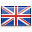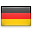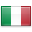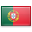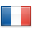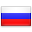# Give in a sentence

I give up.
Give it up.
to give in.
give it a go.
I mean give.
Give it time.
give it to us.

Give me warm.
Never give up.
It will give.
Give with joy.
Give up this.
I'd give this.
give it to them.
Give me a call.
Give her a hug.
Give it a shot.
Give with love.
Give it to me.
Do not give up.
give us His own.
and give it back.
Give it meaning.
Give your hair.
If you give of.
Give this up!.
I will give to.
and giving.
By giving a.
Giving me a.
It is giving.
30 giving me a.
In giving him.
By giving heed.
A woman giving.
00, giving me \$1.
By giving this.
I was giving it.
of a giving heart.
giving her or why.
Giving up ground.
the act of giving.
So try giving it.
After giving the.
Of my giving away.
God is giving them.
way of giving back.
I remember giving.
After giving each.
Giving to the Poor.
giving in to others.
Instead of giving.
giving me the chills.
Giving Sue a quick.
After giving him a.
After giving birth.
Giving to the Needy.
Giving her hus-.
That is a given.
was given to it.
in a given year.
As given under.
I was given a.
Has been given.
That is given.
It’s a given.
Being given out.
An F flat given.
Cal had given me.
I was given.
at any given time.
We’d given in.
Is already given.
Given the chance.
order he was given.
I have given you.
Given that I had.
If given in reply.
given true C# self.
Given that Spain.
And it was given.
He had given the.
The gesture given.
Given such stead-.
God has given all.
God has given man.
and given to others.
It gives it.
He gives His.
It gives you.
He gives the.
gives it to us.
It gives the.
it gives a man.
This gives a.
He gives you.
Only gives up.
And gives the.
This gives us.
Than he gives.
It gives us a.
It gives me.
It gives me an.
It gives you a.
This gives us2.
What gives the.
This gives us a.
the 5 & 3 gives 1.
She gives you a.
If she gives me.
It gives you no.
and gives them an.
This gives reason.
And she gives him.
He gives me a.
Soon, he gives up.
6 I gave.
He gave up.
He gave a.
I gave them.
She gave a.
gave it to me.
He gave man.
It gave one.
And gave the.
That gave me.
It gave knew.
What gave it.
Janine gave a.
And they gave.
But he gave me.
Then she gave.
11 And I gave.
He gave His Son.
Eke just gave in.
and gave it up.
gave a low growl.
The smoke gave.
He sort of gave.
that you gave me.
She gave hers too.
Burton gave the.
She gave me love.
She gave us the.
She gave it back.
Godfrey gave him.